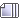dupaAuthor Topic: the robots are coming get your a.i. brains ready !  (Read 145474 times)

ArtRe: the robots are coming get your a.i. brains ready !
« Reply #615 on: September 22, 2019, 09:58:16 pm »
If he would have added that last 9 to the sum of 1 through 8 (36) he would have gotten 45 or which 4 + 5 = 9. No escaping it.

In fact, ANY number when multiplied by 9 will reduce to...you guessed it...9.

Do the multiplication tables for 9 and then add the sum together...yep 9.

3584792 * 9 = 32,263,128 = 3+2+2+6+3+1+2+8 = 27 = 2+7 = 9

11123 * 9 = 100107 = 1+0+0+1+0+7 = 9

The mystic power of 9 boys and girls...

Nice one Cyber!
In the world of AI it's the thought that counts!

- Art -